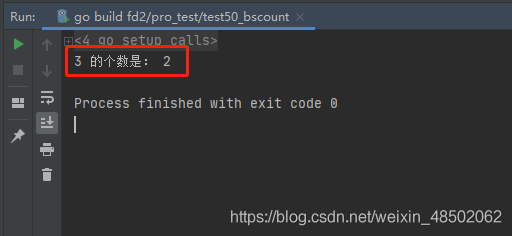November 11, 2020: handwritten code: how to get the number of specified elements in an ordered array?

november handwritten code number specified

1. Ergodic method . There is no code .
2. Dichotomy . Binary search element , And then look for the left boundary , Look for the right boundary again , Finally, subtracting the left boundary from the right boundary is to specify the number of elements . This problem is actually a combination of the following three questions .

1. In an ordered array , Find out if a number exists .
2. In an ordered array , look for >= The left most position of a number .
3. In an ordered array , look for <= On the far right .

golang The code is as follows ：

package main
import "fmt"
func main() {

arr := []int{
0, 1, 2, 3, 3, 5, 5, 5, 5, 5, 5, 5, 5, 5, 6, 7, 8}
v := 3
fmt.Println(v, " The number of is ：", BSCount(arr, v))
}
// Dichotomy
func BSCount(arr []int, v int) int {

L := 0
R := len(arr) - 1
M := -1
// L..R
mid := -1
// Find the target value and narrow the left margin L And the right border R The scope of the
for L <= R {

mid = L + (R-L)>>1
if arr[mid] == v {

M = mid
break
} else if arr[mid] > v {

R = mid - 1
} else {

L = mid + 1
}
}
// No target value found , Go straight back to
if M == -1 {

return 0
}
index := 0
LL := L // Cache the original left boundary
RR := R // Cache the original right boundary
// Find the left border
R = M // Narrow the scope of
for L <= R {

mid = L + (R-L)>>1
if arr[mid] >= v {

index = mid
R = mid - 1
} else {

L = mid + 1
}
}
LL = index // The left boundary is set
R = RR // The original right boundary has changed , It needs to be restored to the previous borders
// Find the right border
L = M // Narrow the scope of
for L <= R {

mid = L + (R-L)>>1
if arr[mid] <= v {

index = mid
L = mid + 1
} else {

R = mid - 1
}
}
RR = index // The right boundary is determined
return RR - LL + 1
}

The results are as follows ：